Chi-Square Test Calculator for Variance

Target: To check if the assumed σ20 is statistically correct, based on a sample varaince S2

Example1: A farmer calculated last year the varaince of the apples' weight in his apple orchard σ20 equals 2 kg2, based on big sample
Current year he checked a small sample of apples and the sample varaince S2 equals 3 kg2
Was the variance of the apple's weight changed this year?

Assumptions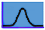The population's distribution is Normal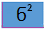You assume you know the population variance ( σ2=σ20)

Required Sample Data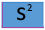Sample variance of the population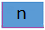Sample size of the population

Enter input data and then press "Calculate test" button to get the results

Hypotheses

 H0: σ2 ≥ σ20 H0: σ2 = σ20 H0: σ2 ≤ σ20 H1: σ2 < σ20 H1: σ2 ≠ σ20 H1: σ2 > σ20

Test statisticχ² distribution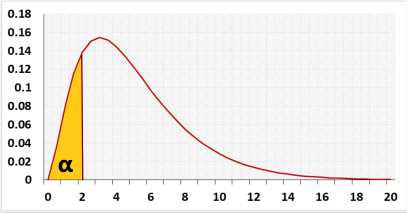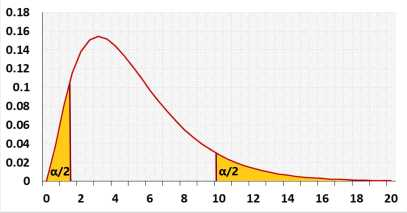Test calculation

If you enter raw data, the tool will run the Shapiro-Wilk normality test and calculate outliers, as part of the test calculation.

Statistic Data

 α: Significant level (0-1), maximum chance allowed rejecting H0 while H0 is correct (Type1 Error) Outliers: IncludedExcluded It is not recommended to exclude outliers unless you know the reasons
or or enter summarized data (x, n, σ, S) in Group form

Enter sample data

Header: You may change groups' name to the real names.
Data: When entering data, press Enter after each value

It is okay to leave empty cells, empty cells or non numeric cells won't be counted

Enter sample data

You may copy data from Excel, Google sheets or any tool that separate data with Tab and Line Feed.
Copy the data, one column includes the header, and paste below.

It is okay to leave empty cells, empty cells or non numeric cells won't be counted

Name:

 n: Sample size σ0: Standard deviation S: Sample Standard deviation skewness: Normality pval: Shapiro Wilk test Outliers count: ,based on the Tukey's fences method, k=1.5

validation message